# 1 Introduction

The main objective of the CancerInSilico package is to provide an R interface for running mathematical models of tumor progression. In C++, we have implemented an off-lattice, cell-based model from Drasdo and Höhme (2003) and this package provides the neccesary R functions to run the C++ code and analyze the output in R. In general, cell-based models simulate each cell in a tumor, accounting for interactions with its neighbors and its local environment. This model specifically models cell-cycle, intercellular forces, and cellular motility. By doing so, it places focus on the cell-cell dynamics which allows for a detailed exploration of intra- and inter-cellular mechanisms that effect overall population dynamics of cells in a tumor.

We note that the mathematical model from Drasdo and Höhme (2003) is one of a larger class of cell-based models of tumor progression (see Szabo and Merks 2013 review). With this in mind, we have developed the package CancerInSilico so that additional types of cell-based models can be easily integrated. Future versions of the package will focus on expanding the types of models available to the user.

# 2runCancerSim: Main function to simulate tumor progression

The runCancerSim function provides a centralized R interface to run c++ code for cell-based models of tumor progression, released with this package. The following code provides an example of running CancerInSilico to simulate clonal outgrowth from a single cell with the Drasdo and Höhme (2003) model over 84 hours using the central model simulation function runCancerSim.

single_cell_run <- runCancerSim(initialNum = 1, runTime = 84)
plotCells(single_cell_run, 84)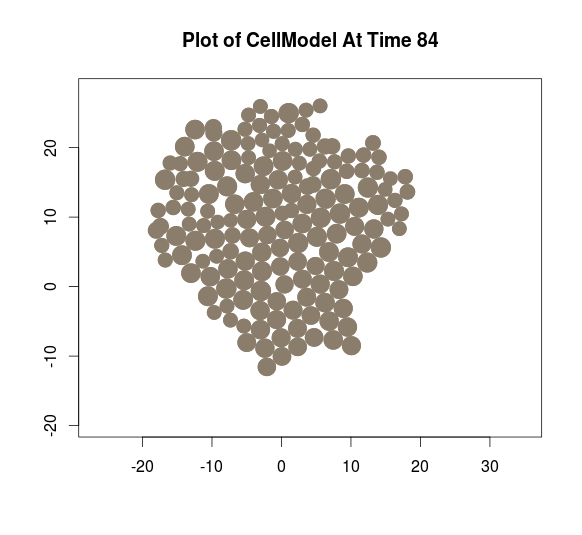Figure 1 the distribution of cells from this simulation at the final 84 hour time point using the plotting function plotCells, described further in Section 2.3.5.

## 2.1runCancerSim Usage

runCancerSim(initialNum,
runTime,
density = 0.1,
cycleLengthDist = 12,
inheritGrowth = F,
outputIncrement = 6,
randSeed = 0,
modelType = "DrasdoHohme2003",
...)

## 2.2 Parameters

### 2.2.1 Parameter Overview

initialNum - [integer] required parameter, must be greater than zero
runTime - [double] required parameter, must be greater than zero
density - [double; default = 0.1] optional parameter, must be in the range (0,0.1)
cycleLengthDist - [array of doubles; default = 12] optional parameter, all values must be greater than 2
inheritGrowth - [bool; default = FALSE] optional parameter
outputIncrement - [double; default = 6.0] optional parameter, must be greater than 0.1
randSeed - [double; default = 0.0] optional parameter
modelType - [string; default=“DrasdoHohme2003”] optional parameter

### 2.2.2 Parameter Desciption

initialNum

The number of cells in the model at time zero. A value of 1 simulates growth from a single cell.

runTime

The number of (model) hours to run the simulation for. For an explanation of the time scale in the model, see the description for cycleLengthDist.

density

The cells are initially seeded randomly throughout a circle, whose radius is calculated based on the density parameter. density in this case is defined as the ratio of the total area of the cells and the area of the circle. Providing a density higher than 0.1 will result in an error, since the program cannot efficiently seed cells that close together.

cycleLengthDist

The model does not have a length scale so the dimensions of the cells are arbitrary, but it does have a time scale - set by this parameter. The value passed in cycleLengthDist is the average length (in terms of simulation hours) of the cell-cycle for a cell in isolation. If a vector is passed in cycleLengthDist then it is treated as a distribution of times and each cell is assigned a random value (uniform) from this vector when it is born. Cells are initially seeded at random points within the cell-cycle.

inheritGrowth

Cells have the option of inheriting the cycle-length from their parent or sampling from cycleLengthDist when they are born. This allows for “growth” rates to be affected in a heritable way (i.e. simulate a mutation by setting a small proportion of cycle-lengths to be extremely short and set inheritGrowth to TRUE)

outputIncrement

The amount of (model) hours in between each display during the run.

randSeed

The user can pass in a random seed of their choosing. All random samples in the model will be affected by this seed. The default seed is 0.

modelType

String indicating which cell-based model is run. By default, it is set to “DrasdoHohme2003” to simulate cells with the c++ code for the Drasdo and Höhme (2003) implemented in the src directory.

### 2.2.3Drasdo and Höhme (2003) Specific Parameters

epsilon - [double; default = 10.0] optional parameter, must be greater than zero
nG - [double; defualt = 24.0] optional parameter, must be greater than one

In the Drasdo and Höhme (2003) model, cells primarily do two things: move and grow. When a cell grows to twice its original volume, it divides. This process happens at a rate depending on what the user sets cycleLengthDist to be. Cells move in order to arrange themselves to respect the intercellular forces mentioned in the introduction. High values for epsilon make it less likely for cells to move against the intercelluar forces. High values for nG give the model more time in between growth steps to find the ideal configuration based on the present intercellular forces. These parameters are described in more detail in Drasdo and Höhme (2003)

## 2.3 Output Variables and CellModel class

The output of the model is stored in a CellModel object. This object has a field containing all cell data, mCells, and a field for each parameter. To get a named list of all the parameters users can call getParameters on a CellModel object. mCells is a list that contains information about the cell population at every time step in the model. The primary purpose of this object is to store all the information about a run in a single place and provide a standard data structure for the package functions to handle the raw data safely.

# the result of runCancerSim stored in
# basic_sim are a CellModel object
basic_sim <- runCancerSim(initialNum = 3,
runTime = 48,
density = 0.1,
cycleLengthDist = 10 + rexp(1000,1/4),
inheritGrowth = FALSE,
outputIncrement = 6,
randSeed = 10,
epsilon = 10,
nG = 6)
## time = 0.00
## size = 3
## time = 6.00
## size = 5
## time = 12.00
## size = 6
## time = 17.99
## size = 8
## time = 23.99
## size = 11
## time = 29.99
## size = 15
## time = 35.99
## size = 22
## time = 41.98
## size = 24
## time = 47.98
## size = 36
## time = 48.00
## size = 36

## 2.4 Functions for analysis of CellModel class

The following describes several functions used to get relevant information from the CellModel object in a user-friendly format, without requiring direct interaction with the complex fields from raw data stored in the CellModel class.

### 2.4.1show

This shows the parameters of a model as well as all available function in the CellModel class.

Function Signature:

show(model)

Arguments:

model - A CellModel object

Return:

shows all parameters and available functions for the CellModel object

Example:

## example call
show(basic_sim) 
## model parameters:$initialNum ##  3 ## ##$runTime
##  48
##
## $initialDensity ##  0.1 ## ##$inheritGrowth
##  FALSE
##
## $outputIncrement ##  6 ## ##$randSeed
##  10
##
## $epsilon ##  10 ## ##$nG
##  6
##
## $timeIncrement ##  0.003222746 ## ##$cycleLengthDist
##  13.91716
##
## available functions:
## interactivePlot
## plotCells
## getParameters
## getDensity
## getCycleLengths
## getNumberOfCells

### 2.4.2getParameters

This is the function used to get any information about the parameters passed into the model.

Function Signature:

getParameters(model, fullDist = FALSE)

Arguments:

model - A CellModel object
fullDist - TRUE/FALSE

Return:

returns a named list containing the parameters for model. If fullDist is TRUE it returns the entire vector for cycleLengthDist, otherwise it just returns the mean.

Example:

## example call
getParameters(basic_sim) 
## $initialNum ##  3 ## ##$runTime
##  48
##
## $initialDensity ##  0.1 ## ##$inheritGrowth
##  FALSE
##
## $outputIncrement ##  6 ## ##$randSeed
##  10
##
## $epsilon ##  10 ## ##$nG
##  6
##
## $timeIncrement ##  0.003222746 ## ##$cycleLengthDist
##  13.91716

In order to see the full distribution that was passed in, it is neccesary to specifiy:

d <- getParameters(basic_sim, fullDist=TRUE)$cycleLengthDist plot(density(d),main="Cycle Length Distribution - Parameter",xlab="length")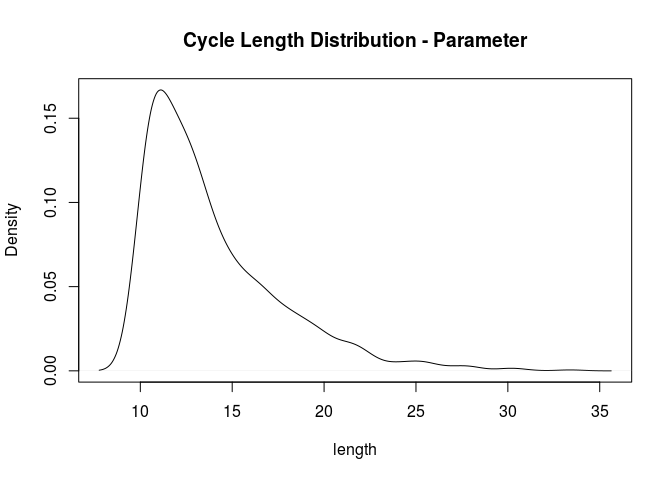### 2.4.3getNumberOfCells Function Signature: getNumberOfCells(model, time) Arguments: model - A CellModel object time - A double between 0 and the runTime in model Return: The number of cells in model at time. Example: length <- getParameters(basic_sim)$runTime
t <- sapply(0:length,getNumberOfCells,model=basic_sim)
plot(0:length, t, type = "l", xlab="time", ylab="# of cells")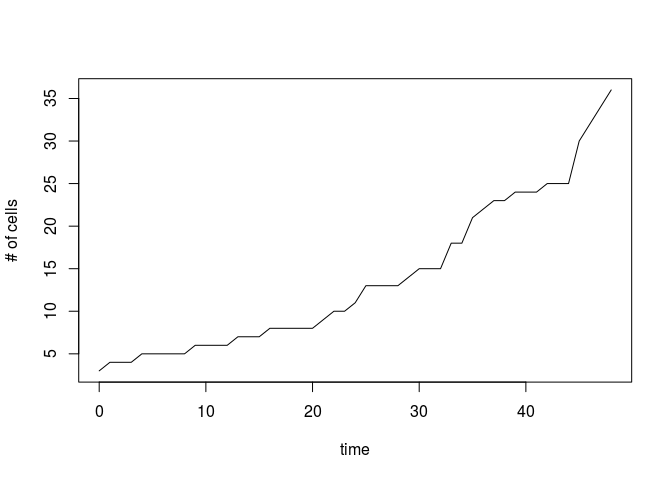### 2.4.4getCycleLengths

Function Signature:

getCycleLengths(model, time)

Arguments:

model - A CellModel object
time - A double between 0 and the runTime in model

Return:

A vector of cell-cycle lengths for each cell in model at time.

Example:

cyc_len <- getCycleLengths(basic_sim,0)
plot(density(cyc_len),main="Cycle Length Distribution - Time 0",xlab="length")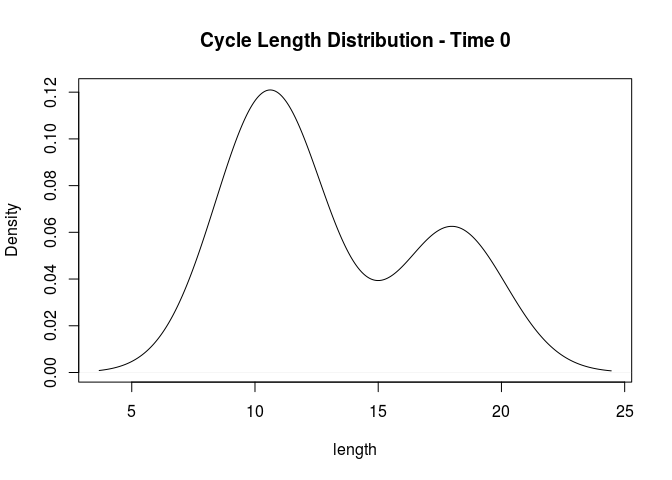### 2.4.5getDensity

Function Signature:

getDensity(model, time)

Arguments:

model - A CellModel object
time - A double between 0 and the runTime in model

Return:

The density of cells in model at time

Example:

den <- sapply(0:length,getDensity,model=basic_sim)
plot(0:length,den,type="l",xlab="time",ylab="cell density")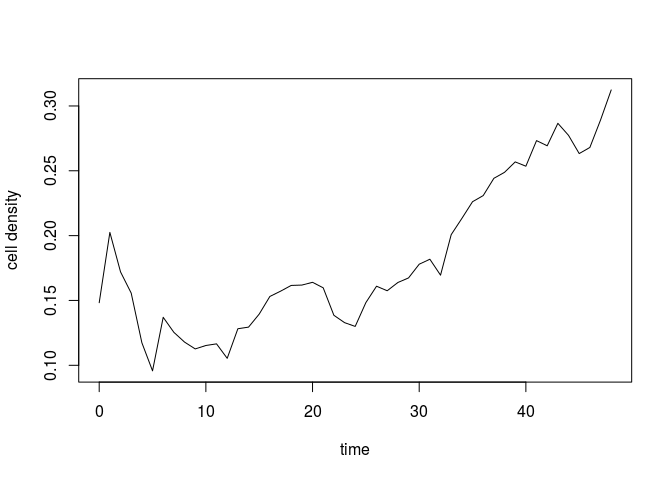### 2.4.6plotCells

Function Signature:

plotCells(model, time)

Arguments:

model - A CellModel object
time - A double between 0 and the runTime in model

Return:

plots a visual representation of the cells at time.

Example:

plotCells(basic_sim,0)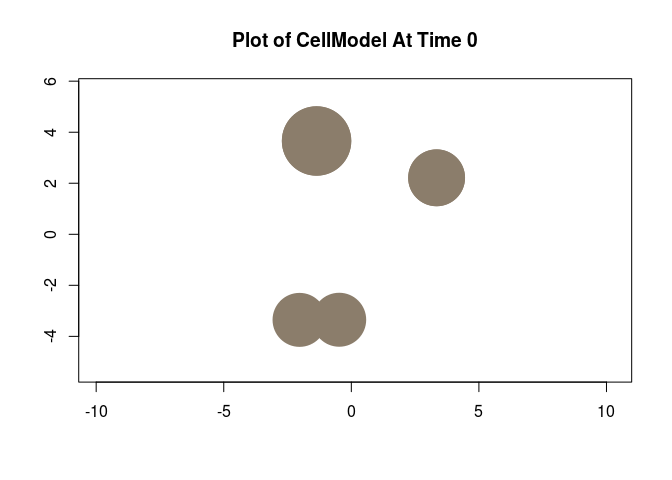plotCells(basic_sim,length)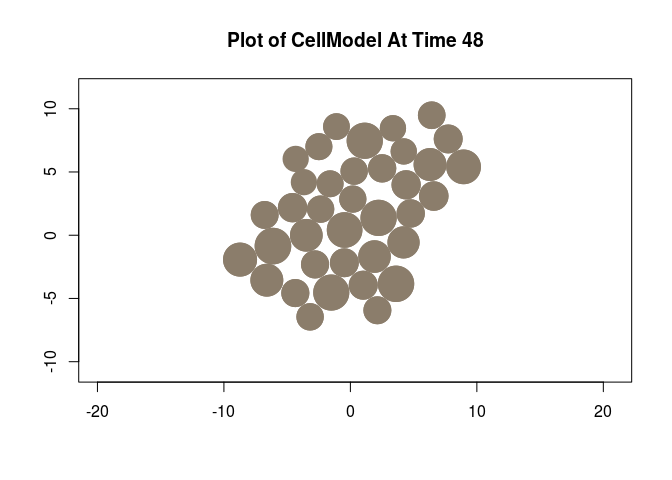### 2.4.7interactivePlot

The interactivePlot function is similiar to plotCells except there are additional commands that allow the user to step through the simulation and watch it progress as well as display information at the current timestep.

Function Signature:

interactivePlot(model, time = 0)

Arguments:

model - A CellModel object
time - A double between 0 and the runTime in model

Return:

Calls plotCellsAtTime(model,time). From there the user can input one of the following commands to step through the simulation and observe how the cells change over time.

Basic Commands:
b ARG = back ARG timesteps (default ARG = 1)
n ARG = forward ARG timesteps (default ARG = 1)
h = basic command help